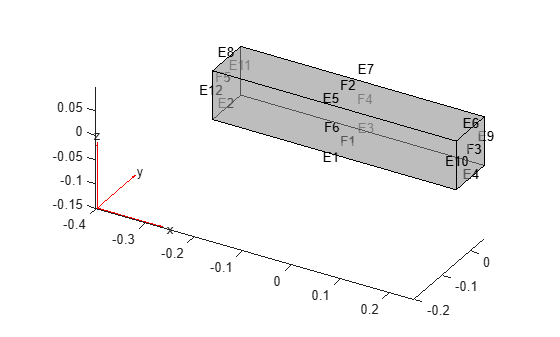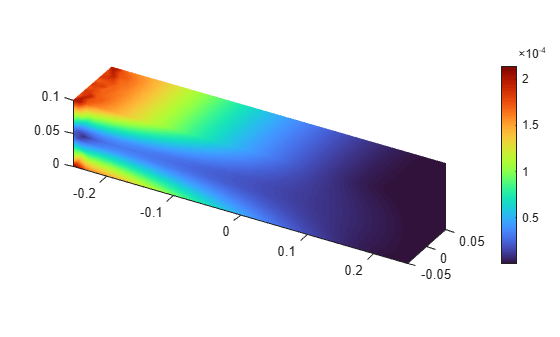# femodel

Finite element analysis model object

Since R2023a

## Description

An `femodel` object contains information about a finite element problem: analysis type, geometry, material properties, boundary conditions, loads, initial conditions, and other parameters.

## Creation

### Syntax

``model = femodel(AnalysisType=analysistype)``
``model = femodel(AnalysisType=geometry,Geometry=Geometry)``

### Description

example

````model = femodel(AnalysisType=analysistype)` creates a finite element analysis model for the specified analysis type.```

example

````model = femodel(AnalysisType=geometry,Geometry=Geometry)` also assigns the specified geometry object to its `Geometry` property.```

### Input Arguments

expand all

Type of problem, specified as one of these values:

• `"structuralStatic"` creates a model for static structural analysis.

• `"structuralModal"` creates a model for modal structural analysis.

• `"structuralTransient"` creates a model for transient structural analysis.

• `"structuralFrequency"` creates a model for frequency response structural analysis.

• `"thermalSteady"` creates a model for steady-state thermal analysis.

• `"thermalModal"` creates a model for modal thermal analysis.

• `"thermalTransient"` creates a model for transient thermal analysis.

• `"electrostatic"` creates a model for electrostatic analysis.

• `"magnetostatic"` creates a model for magnetostatic analysis.

• `"electricHarmonic"` creates a model for harmonic electromagnetic analysis with an electric field type.

• `"magneticHarmonic"` creates a model for harmonic electromagnetic analysis with a magnetic field type.

• `"dcConduction"` creates a model for DC conduction analysis.

This argument sets the AnalysisType property.

Data Types: `char` | `string`

Geometry description, specified as an `fegeometry` object. This argument sets the Geometry property.

## Properties

expand all

Type of problem, specified as `"structuralStatic"`, `"structuralModal"`, `"structuralTransient"`, `"structuralFrequency"`, `"thermalSteady"`, `"thermalModal"`, `"thermalTransient"`, `"electrostatic"`, `"magnetostatic"`, `"electricHarmonic"`, `"magneticHarmonic"`, or `"dcConduction"`.

This property is set by the analysistype input argument.

Data Types: `string`

Geometry description, specified as an `fegeometry` object. This property is set by the geometry input argument.

Type of two-dimensional problem, specified as `"planeStress"`, `"planeStrain"`, or `"axisymmetric"`. This property does not apply to models with 3-D geometries.

Data Types: `string`

Material properties, specified as a `1`-by-`N` array of `materialProperties` objects. Here, `N` is the number of faces in a 2-D geometry or number of cells in a 3-D geometry. Each `materialProperties` object contains material properties for one face or cell of the geometry.

## Boundary Conditions

Boundary conditions on geometry faces, specified as a `1`-by-`N` array of `faceBC` objects. Here, `N` is the number of faces in a geometry. Each `faceBC` object contains boundary conditions for one face of the geometry.

Boundary conditions on geometry edges, specified as a `1`-by-`N` array of `edgeBC` objects. Here, `N` is the number of edges in a geometry. Each `edgeBC` object contains boundary conditions for one edge of the geometry.

Boundary conditions on geometry vertices, specified as a `1`-by-`N` array of `vertexBC` objects. Here, `N` is the number of vertices in a geometry. Each `vertexBC` object contains boundary conditions for one vertex of the geometry.

Settings for modeling thermal radiation between surfaces without conductive media, specified as a `surfaceToSurfaceSettings` object. The default empty value results in the surface-to-ambient solution.

Loads on geometry cells, specified as a `1`-by-`N` array of `cellLoad` objects. Here, `N` is the number of cells in a geometry. Each `cellLoad` object contains loads for one cell of the geometry.

Loads on geometry faces, specified as a `1`-by-`N` array of `faceLoad` objects. Here, `N` is the number of faces in a geometry. Each `faceLoad` object contains loads for one face of the geometry.

Loads on geometry edges, specified as a `1`-by-`N` array of `edgeLoad` objects. Here, `N` is the number of edges in a geometry. Each `edgeLoad` object contains loads for one edge of the geometry.

Loads on geometry vertices, specified as a `1`-by-`N` array of `vertexLoad` objects. Here, `N` is the number of vertices in a geometry. Each `vertexLoad` object contains loads for one vertex of the geometry.

## Initial Conditions

Initial conditions on geometry cells, specified as a `1`-by-`N` array of `cellIC` objects. Here, `N` is the number of cells in a geometry. Each `cellIC` object contains initial conditions for one cell of the geometry.

Initial conditions on geometry faces, specified as a `1`-by-`N` array of `faceIC` objects. Here, `N` is the number of faces in a geometry. Each `faceIC` object contains initial conditions for one face of the geometry.

Initial conditions on geometry edges, specified as a `1`-by-`N` array of `edgeIC` objects. Here, `N` is the number of edges in a geometry. Each `edgeIC` object contains initial conditions for one edge of the geometry.

Initial conditions on geometry vertices, specified as a `1`-by-`N` array of `vertexIC` objects. Here, `N` is the number of vertices in a geometry. Each `vertexIC` object contains initial conditions for one vertex of the geometry.

## Other Parameters

Mass proportional damping, specified as a nonnegative number.

Data Types: `double`

Stiffness proportional damping, specified as a nonnegative number.

Data Types: `double`

Reference temperature for a thermal load, specified as a real number. The reference temperature corresponds to the state of zero thermal stress of the model. The value 0 implies that the thermal load is specified in terms of the temperature change and its derivatives.

To specify the reference temperature for a thermal load in your model, assign the property value directly, for example, ```model.ReferenceTemperature = 10```. To specify the thermal load itself, use `cellLoad`.

Data Types: `double`

Algorithm options for the PDE solvers, specified as a `PDESolverOptions` object. The properties of `PDESolverOptions` include absolute and relative tolerances for internal ODE solvers, maximum solver iterations, and so on. For a complete list of properties, see PDESolverOptions Properties.

## Constants

Permittivity of vacuum for the entire model, specified as a positive number. This value must be consistent with the units of the model. If the model parameters are in the SI system of units, then the permittivity of vacuum must be `8.8541878128e-12`.

Data Types: `double`

Permeability of vacuum for the entire model, specified as a positive number. This value must be consistent with the units of the model. If the model parameters are in the SI system of units, then the permeability of vacuum must be `1.2566370614e-6`.

Constant of proportionality in Stefan-Boltzmann law governing radiation heat transfer, specified as a positive number. This value must be consistent with the units of the model. Values of the Stefan-Boltzmann constant in commonly used systems of units are:

• SI —`5.670367e-8` W/(m2·K4)

• CGS— `5.6704e-5` erg/(cm2·s·K4)

• US customary — `1.714e-9` BTU/(hr·ft2·R4)

Data Types: `double`

## Object Functions

 `generateMesh` Create triangular or tetrahedral mesh `pdegplot` Plot PDE geometry `pdemesh` Plot PDE mesh `solve` Solve structural analysis, heat transfer, or electromagnetic analysis problem

## Examples

collapse all

Create a model for solving a thermal transient problem.

`model = femodel(AnalysisType="thermalTransient")`
```model = 1x1 femodel array Properties for analysis type: thermalTransient AnalysisType: "thermalTransient" Geometry: [0x0 fegeometry] PlanarType: "" MaterialProperties: [0x0 materialProperties] Boundary Conditions FaceBC: [0x0 faceBC] EdgeBC: [0x0 edgeBC] VertexBC: [0x0 vertexBC] ThermalRadiation: [] Loads CellLoad: [0x0 cellLoad] FaceLoad: [0x0 faceLoad] EdgeLoad: [0x0 edgeLoad] VertexLoad: [0x0 vertexLoad] Initial Conditions CellIC: [0x0 cellIC] FaceIC: [0x0 faceIC] EdgeIC: [0x0 edgeIC] VertexIC: [0x0 vertexIC] Other Parameters DampingAlpha: 0 DampingBeta: 0 ReferenceTemperature: [] SolverOptions: [1x1 pde.PDESolverOptions] Constants StefanBoltzmann: [] Show all properties ```

Create a model for solving a static plane-strain structural problem.

Create an `femodel` object for solving a static structural problem, and assign a 2-D geometry to the model.

```model = femodel(AnalysisType="structuralStatic", ... Geometry="PlateHolePlanar.stl")```
```model = 1x1 femodel array Properties for analysis type: structuralStatic AnalysisType: "structuralStatic" Geometry: [1x1 fegeometry] PlanarType: "planeStress" MaterialProperties: [0x1 materialProperties] Boundary Conditions FaceBC: [0x1 faceBC] EdgeBC: [0x5 edgeBC] VertexBC: [0x5 vertexBC] Loads FaceLoad: [0x1 faceLoad] EdgeLoad: [0x5 edgeLoad] VertexLoad: [0x5 vertexLoad] Other Parameters ReferenceTemperature: [] SolverOptions: [1x1 pde.PDESolverOptions] Show all properties ```

By default, `femodel` assumes that a 2-D problem is a plane-stress problem. Specify the plane-strain problem type.

```model.PlanarType = "planeStrain"; model.PlanarType```
```ans = "planeStrain" ```

Create a geometry of a beam.

`gm = multicuboid(0.5,0.1,0.1);`

Plot the geometry.

```pdegplot(gm,FaceAlpha=0.5, ... FaceLabels="on", ... EdgeLabels="on")```Create an `femodel` object for solving a static structural problem, and assign the geometry to the model.

```model = femodel(AnalysisType="structuralStatic", ... Geometry=gm)```
```model = 1x1 femodel array Properties for analysis type: structuralStatic AnalysisType: "structuralStatic" Geometry: [1x1 fegeometry] MaterialProperties: [0x1 materialProperties] Boundary Conditions FaceBC: [0x6 faceBC] EdgeBC: [0x12 edgeBC] VertexBC: [0x8 vertexBC] Loads CellLoad: [0x1 cellLoad] FaceLoad: [0x6 faceLoad] EdgeLoad: [0x12 edgeLoad] VertexLoad: [0x8 vertexLoad] Other Parameters ReferenceTemperature: [] SolverOptions: [1x1 pde.PDESolverOptions] Show all properties ```

Specify Young's modulus, Poisson's ratio, and the mass density.

```model.MaterialProperties = materialProperties(YoungsModulus=210e3, ... PoissonsRatio=0.3, ... MassDensity=2.7e-6); model.MaterialProperties```
```ans = 1x1 materialProperties array Properties for analysis type: structuralStatic Index CTE PoissonsRatio YoungsModulus MassDensity ShearModulus 1 [] 0.3000 210000 2.7000e-06 [] Show all properties ```

Specify a gravity load on the beam.

```model.CellLoad = cellLoad(Gravity=[0;0;-9.8]); model.CellLoad```
```ans = 1x1 cellLoad array Properties for analysis type: structuralStatic Index Gravity AngularVelocity Temperature 1 [0 0 -9.8000] [] [] Show all properties ```

Specify that face 5 is a fixed boundary.

```model.FaceBC(5) = faceBC(Constraint="fixed"); model.FaceBC```
```ans = 1x6 faceBC array Properties for analysis type: structuralStatic Index Constraint XDisplacement YDisplacement ZDisplacement 1 [] [] [] [] 2 [] [] [] [] 3 [] [] [] [] 4 [] [] [] [] 5 fixed [] [] [] 6 [] [] [] [] Show all properties ```

Generate a mesh and assign the result to the model. This assignment updates the mesh stored in the `Geometry` property of the model.

`model = generateMesh(model);`

Solve the model.

`R = solve(model)`
```R = StaticStructuralResults with properties: Displacement: [1x1 FEStruct] Strain: [1x1 FEStruct] Stress: [1x1 FEStruct] VonMisesStress: [7888x1 double] Mesh: [1x1 FEMesh] ```

Plot the von Mises stress.

`pdeviz(R.Mesh,R.VonMisesStress)````ans = PDEVisualization with properties: MeshData: [1x1 FEMesh] NodalData: [7888x1 double] MeshVisible: off Transparency: 1 Position: [0.1300 0.1100 0.6733 0.8150] Units: 'normalized' Use GET to show all properties ```

## Version History

Introduced in R2023a

expand all